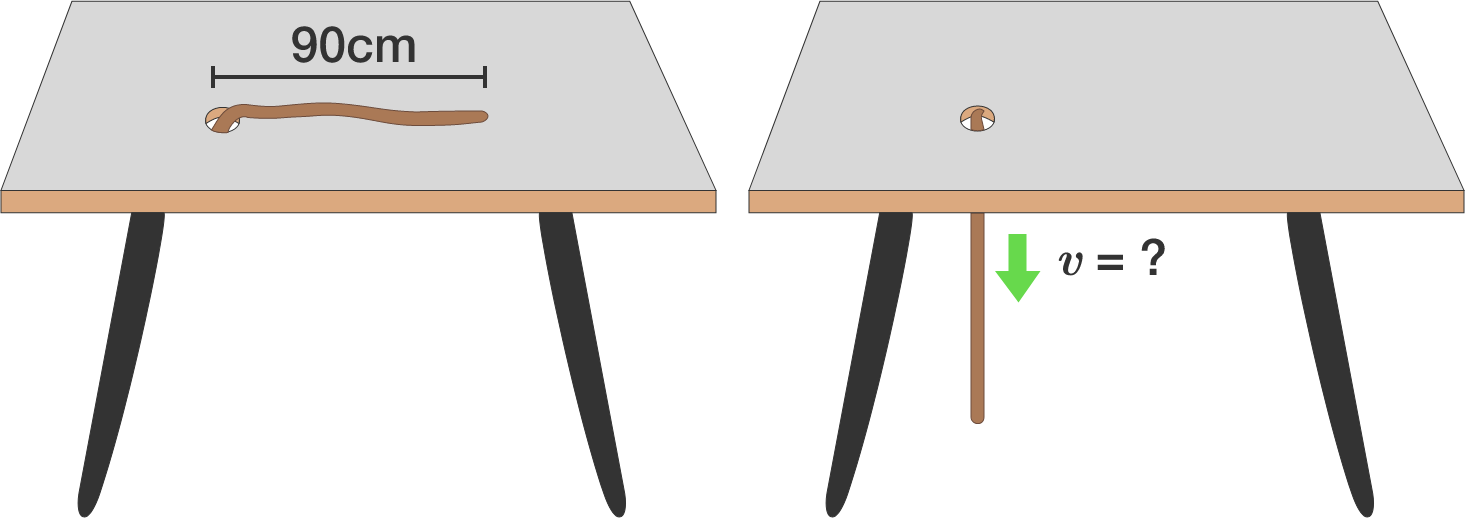# Rope through holeA rope of length $\SI{90}{\centi\meter}$ lies in a straight line on a frictionless table, except for a very small piece at one end which hangs down through a hole in the table.

This piece is released, and the rope slides down through the hole. What is the speed $($in $\text{m/s})$ of the rope (to 2 decimal places) at the instant it loses contact with the table?

Details: $g = \SI[per-mode=symbol]{9.81}{\meter\per\second\squared}.$

×